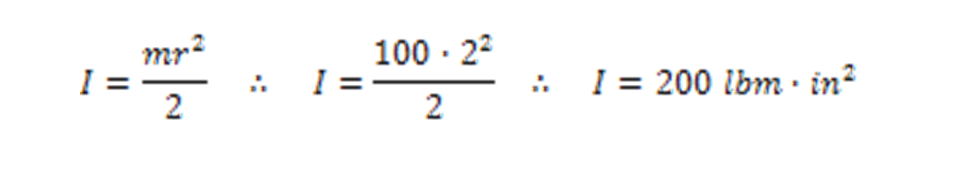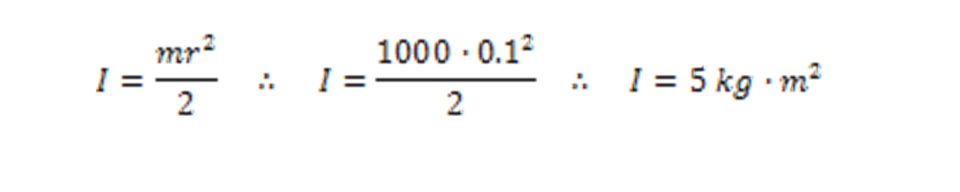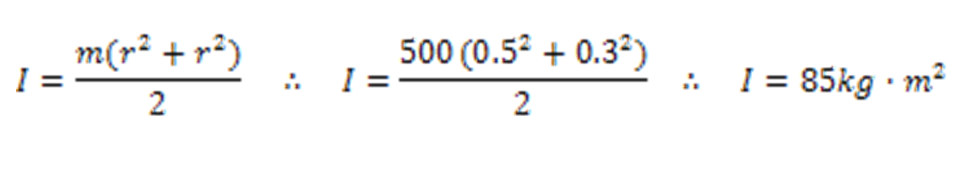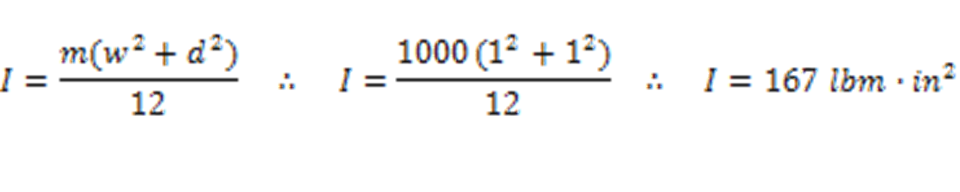This is where you give the visitor a brief introduction to both this blog and your company. Keep the intro pithy and punchy.

# BlogCALCULATING MOMENT OF INERTIA

As a manufacturer of safety couplings and torque limiters, we are often asked to provide some assistance in calculating the moment of inertia of various loads, in order to aid in selecting the appropriate disengagement torque settings.  Once the driving and driven inertia values are known, acceleratin and deceleration rates are used to estimate safety coupling torque adjustment ranges.

Moment of inertia can be described as a mechanical property of the mass of a solid object that quantifies the required torque needed to change the angular velocity of the object about an axis. This inertia is also known as the mass moment of inertia, first moment, or, rotational inertia.  This moment can be used to calculate how much energy it takes to get an object rotating which is especially useful in mechanical drive lines with motors and engines.  When sizing a torque limiter or safety coupling, it is important that the disengagement torque value be set to a value higher than what is required to accelerate the load up to speed.  It is also helpful to be aware of the torque levels resulting from abruptly decelerating a rotating mass, which is where torque limiters and safety couplings come into play, protecting driveline components from the excessive torques resulting from an unintended blockage stopping a machine too rapidly.  Moment of inertia often denoted as (I) is a unit of mass multiplied by area squared (M*A2). In the case of a two inch diameter rotating shaft with a mass of one hundred pounds, moment of inertia can be calculated per the example below.Calculating the moment of inertia for a 0.1 meter diameter shaft with a mass of 1000 kg is done in the exact same manner.Finding the moment of inertia for a tube or hollow shaft is very similar. In the next example, we will find the value for a 0.5 meter outside diameter tube with a 0.3 meter inside diameter. The mass of the tube is 500 kg.The calculation for a moment of a square or rectangular shaft or shape can also be useful. In this example, the moment of a one inch by one inch square shaft with a mass of 1000 pounds will be found.The examples above are handy for calculations involving sizing of mechanical drive components, and not just safety couplings. Inertia ratios of rotating shafts and motor rotors are critical to proper operation of many drives. These calculations are all done about the axis of typical shaft rotation and cannot be used for a different axis.  It is important to note that this is the mass moment and the calculation gives us a property of mass at a distance from the axis of rotation. As with all rotating objects, the moment increases exponentially as a mass moves away from the axis of rotation.  These basic facts of physics are critical for machine design and have been a primary guiding principle for centuries of mechanical engineering.